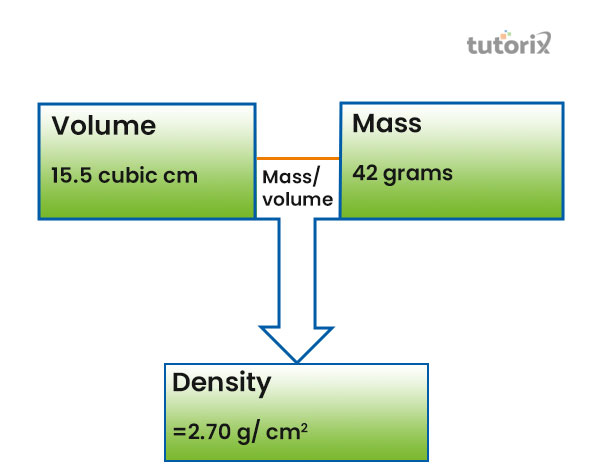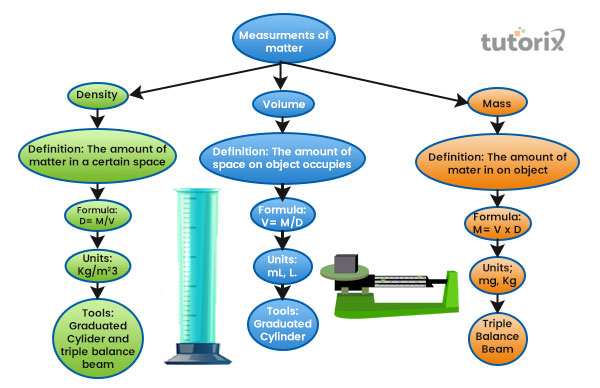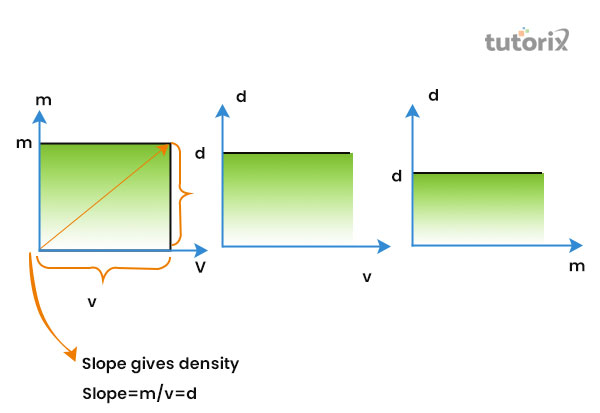# Relation Between Density and Volume

## Introduction

Any object on the earth includes the most basic characteristics such as volume and density. Both of the properties state vital information about an object that is included the resisting acceleration and the gravitational force.

Additionally, density is computed by separating an entity's mass by its volume. Density is less instinctive than volume or mass. In this tutorial, detailed information regarding density and volume has been elaborate with their connection.Figure 1: Connection between Volume and Density

## Volume

Volume is measured to comprehend the occupied space of an object which can be solid, liquid, or even gas.

A consistent surface, like the space is inhabited or controlled by a sense, can encompass a specific quantity of three-dimensional area (liquid, gas, solid, or plasma). The container's volume is generally believed to symbolise its capability, or the quantity of fluid (liquid or gas) that it may be carried by it, rather than the quantity of occupied space.

Moreover, the displacement of an object can be utilised in the development of the calculation of the volume of the regular and irregular solid space (Waeleh et al. 2021).

The word V represents the volume of a container and it is used as the calculation of the "three-dimensional shapes".

Examples

For instance, a jar which is contained water can be utilised for measuring the mathematical quantity which help detects the amount of water occupied in the jar.

Moreover,

let's assume, cuboids' has the three dimensions of 8cm x 3cm x 5cm. Then, the volume can be estimated with the formula of V = 8cm x 3cm x 5cm. Hence, the result will be V = 120 cubic centimetres.

## Density

The density of an item displays the denseness of that item in a given space. An item's density is described as its "mass per unit volume". Density is effectively a proportion of how tightly value is packed concurrently. It is an individual property used in physics for a separate entity.

Additionally, ρ is the symbol that describes density or it can also be conveyed by the letter D. further, the density of an item is the proportions of how tightly the item is congested concurrently (Differencebetween, 2022).

It is represented as the "mass per unit volume". In a qualitative word, it indicates how weighty an item is at a consistent volume.

Examples

For each substance, the specific density is taken into consideration by calculating it with the specified units. Moreover, the SI unit of Density is estimated by employing "kilograms per cubic meter (kg/m3)". The primary formula for collecting the density is "ρ = m/v". Hence, it can be illustrated, as density is equalled to mass divided by the volume of an item.Figure 2: Connection between the mass, volume, and density

## Relation between Density and Volume

Every item on the earth has included different properties when it comes to measuring the qualitative values. It signifies that precise a slight modification in density probably causes a modification in the volume of that specific item as well. Likewise, the reversal is also correct, wherein even a small transition in volume will conduct a transition in the density of the specific item (Holt et al. 2022). Alongside, an individual should also be knowledgeable about the sign patterns for every parameter to illustrate the connection in mathematical phrases.Figure 3: Relation between Density and Volume

The formal definition of mass allows users to make a calculator of the energy within the context of putting different measurements. Most of the time, momentum and gravitational force are aligned with the behaviour of an item on the earth.

It has been proved in the past that the mass of an item gets varied on the different planets which work with the gravitational forces. Since gravity is closely identical to the Earth's surface, the weight of an item evolves into a good arrow of mass (Thakur et al. 2021). Enriching and decreasing the quantity of material is estimated by increasing and decreasing the substance of the mass.

## The connection between Mass, Density, and Volume

According to physics, there is an internal relationship between all the different properties of any item which is meant for the estimation of weight, and comprehension of the space occupied by an item on a particular surface. Additionally, "mass, density and volume" are used to comprehend the measurement that is generally taken into consideration for calculating different properties (Sciencing, 2022). Unlike volume and mass, increasing the quantity of material calculated does not improve or lower the density. The modification in the volume is referred to as the transformation in the density, and it is worked as vice versa.

## Conclusion

In this tutorial, the comprehension of the connection between the volume and density has been measured with the accurate formula as possible. Moreover, it has been proved in past, that modifying the temperature affects the values of the volume and density. Volume and density have the most used proportion for calculating an item's specific behaviour on the earth. Additionally, as the v and d are symmetrical to each other as the relation, it seems to have control over each other.

Q1. What is the connection between the "Volume and Density"?

Volume (v) and Density (d) is related via the mass of a particular item. Moreover, the modification in either one's value gets another affected to change. Both of the parameters are balanced with each other.

Q2. What is the connection of density correspondence with temperature?

The relation with the temperature can be viewed with the experimentation with water and ice. The ice, which has a temperature of 0-degree, has less density than the normal water.

Q3. Who have invented the formula of Density?

A "Greek scientist Archimedes" found the declaration of density. It is comfortable to compute the density if a person knows the formula and comprehends the corresponding units.

## References

### Journals

Holt, P., Tuominen, T., Nevalainen, J., Bonamente, M., Kuutma, T., Heinämäki, P., & Tempel, E. (2022). The scaling relation between galaxy luminosity and WHIM density from EAGLE simulations with application to SDSS data. Monthly Notices of the Royal Astronomical Society, 513(3), 3387-3398. Retrieved from: https://arxiv.org/pdf/2203.12770

Thakur, D. C., Singh, J., Varughese, A., & Jose, P. P. (2021). Relation between local density and density relaxation near glass transition in glass forming binary mixture. arXiv preprint arXiv:2102.03070. Retrieved from: https://arxiv.org/pdf/2102.03070

Waeleh, N., Saripan, M. I., Mashohor, S., Musarudin, M., & Ahmad Saad, F. F. (2021). Accelerating Monte Carlo N-particle simulation using source volume definition. Retrieved from: https://inis.iaea.org

Websites